There is 23 people in the group. What if I tell you that the probability that (at least) two of them have birthday at the same day is more than 0.5? You don’t believe? Ok, let’s sort it out!

When comparing peoples’ birthdays, we care about number of all possible combinations of 2, order does not matter to us. This is denoted as

For 23 people it means 253 possible couplings. The group do 253 one-to-one glass clinks during a toast.

Let’s move to the probability. The probability of two people having birthday on different days is

Each person has a known birthday date and leap years are not assumed. The complement for this is the probability of two people having birthday on the same day.

The result in the previous section can be applied to a group of three people. For such group, we can create 3 different pairs to compare (see the section Number of combinations).

We want all three people (A, B, C) to have their birthdays (bA, bB, bC) in different days. We query each pair of people in the group and all must be different.

We assume no dependence between the people (e.g. twins present); then probabilities simply multiply

Each term is equivalent to the situation of group of two people from the previous section. We can replace the event for each specific couple by a general birthday match and write in an exponent.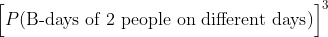Probability expression of the generalized birthday mismatch in a group of 3.

Under our previous assumptions this is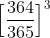Probability value of the generalized birthday mismatch in a group of 3.

Probability of all people in a group of 3 having birthday on different days is 0.9918.

Before generalization onto n people, let’s extend the example for 4 people. In a group of 4 (A, B, C, D) we can create 6 pairs (A-B, A-C, A-D, B-C, B-D, C-D).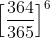Probability value of the generalized birthday mismatch in a group of 4.

The probability of all 4 people having a birthday on different days is 0.9837.

Let’s transform our previous computation for a general group of N people. In the last expression, we replace the exponent with number of combinations for N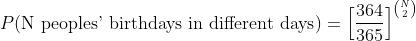Probability expression of the generalized birthday mismatch in a group of N.

The complementary probability can be implemented in Python using this formula.

`>>> # probability distribution for Birthday paradox... bday_paradox = lambda n: 1 — (364/365)**(n*(n-1)/2)`

For different N between 1 and 80 we receive unintuitive results for the probabilities.

`>>> # N in {1,...,80}... import numpy as np... N = np.linspace(1,80,80)... Pn = bday_paradox(N)>>> # plot... import matplotlib.pyplot as plt... fig,ax = plt.subplots(figsize=(10,6))... ax.scatter(N, Pn, s=2)... ax.axhline(1, alpha=.1)... ax.axhline(0, alpha=.1);`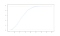Probability distribution of the generalized birthday mismatch in a group of N.

In group of 23 people the probability of somebody having a birthday on the same date is greater than 0.5.

`>>> Pn # indexing from 00.5004771540365807`

To get probability 0.9 we need a group of 41 people.

`>>> np.argmin(Pn < 0.9)41`

In this article, we described a phenomenon called Bithday paradox, which describes surprisingly high probability of two people having birthday on the same day. The probability distribution for the group size is visualized.

In a group of 23 people the probability is more than 0.5.

Hi, I am data scientist, statistician and software developer.

## More from Martin Beneš

Hi, I am data scientist, statistician and software developer.